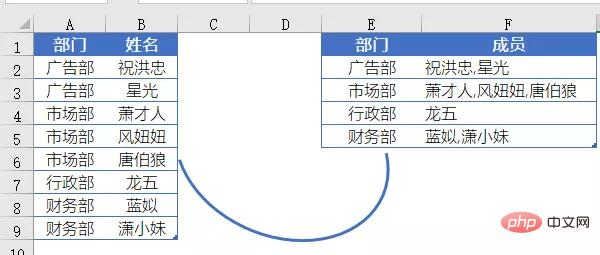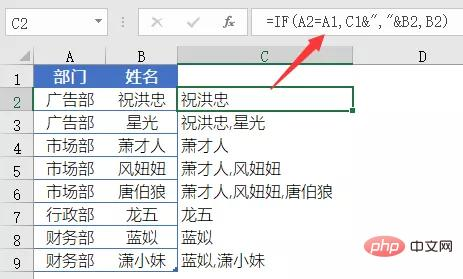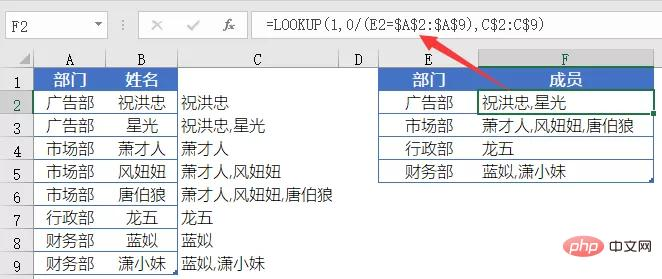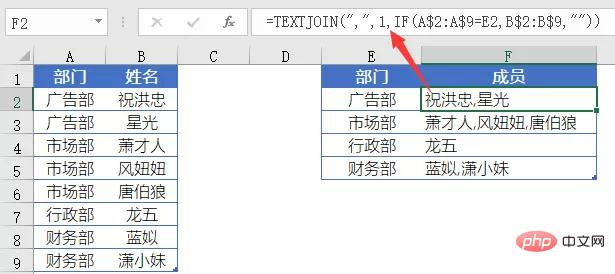# Excel怎样将符合条件的结果放到一个单元格A列是某公司部门名称，B列是人员姓名。

=IF(A2=A1,C1&”,”&B2,B2)F2输入公式：

=LOOKUP(1,0/(E2=\$A\$2:\$A\$9),C\$2:C\$9)C列为辅助列，是一个简单的IF函数。

=IF(A2=A1,C1&”,”&B2,B2)

=IF(A3=A2,C2&”,”&B3,B3)

A3和A2的值相等，返回真值C2&”,”&B3。

C2为上个公式所返回的结果B2（祝洪忠），B3的值是”星光”，所以C3最后结果为”祝洪忠,星光”。

=LOOKUP(1,0/(E2=\$A\$2:\$A\$9),C\$2:C\$9)

LOOKUP的这个套路，忽略错误值，总是取得最后一个符合条件的结果，我们可以总结为：

=LOOKUP(1,0/(条件区域=指定条件),要返回的目标区域)

=TEXTJOIN(“,”,1,IF(A\$2:A\$9=E2,B\$2:B\$9,””))TEXTJOIN函数的用法为：

=TEXTJOIN(间隔符号,要不要忽略空文本,要合并的内容)

IF(A\$2:A\$9=E2,B\$2:B\$9,””)

{“祝洪忠”;”星光”;””;””;””;””;””;””}

TEXTJOIN函数对IF函数得到的内存数组进行合并，第一参数指定使用间隔符号为逗号，第二参数使用1，表示忽略内存数组中的空文本。• 相关标签：Excel
• 相关文章

相关视频